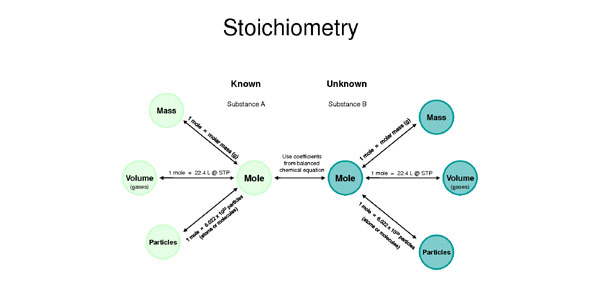10 Questions | Total Attempts: 1302SettingsStoichiometry is the quantitative interpretation of the amount moles of the matter in a chemical reaction. It is also known as the molar bookkeeping of chemistry which means, the study of chemistry and its uses in various fields such as agriculture, pharmaceuticals, food chemistry etc. This quiz has been created to test your knowledge and concepts of stoichiometry. So, let's try out the quiz. All the best!

• 1.
A mole ratio can be the ratio of -
• A.

A reactant to a product

• B.

A reactant to another reactant

• C.

A product to another product

• D.

A product to a reactant

• E.

All of the above

• 2.
How many moles of hydrogen gas are needed to react completely with two moles of nitrogen gas? 3H2 + N2 --> 2NH3
• A.

3 mol

• B.

6 mol

• C.

9 mol

• D.

12 mol

• 3.
How much ammonia (NH3) is produced from two moles of nitrogen gas? 3H2 + N2 --> 2NH3
• A.

8 mol

• B.

6 mol

• C.

4 mol

• D.

2 mol

• 4.
How many moles of oxygen gas are produced from the decomposition of six moles of potassium chloride? 2KClO3 --> 2KCl + 3O2
• A.

9 mol

• B.

3 mol

• C.

6 mol

• D.

10 mol

• 5.
How many moles of potassium chloride are produced from the decomposition of six moles of potassium chloride? 2KClO3 --> 2KCl + 3O2
• A.

2 mol

• B.

3 mol

• C.

6 mol

• D.

7 mol

• 6.
Which of the following has the largest mass of carbon per gram?
• A.

H₂CO

• B.

CH₃CO₂H

• C.

CH₃OH

• D.

CH₃CH₂OH

• 7.
Which of the following statements is true?
• A.

1 mol H atoms = 6.02 X 10²³ H atom

• B.

1 mol pennies = 6.02 X 10²³ pennies

• C.

6.02 X 10²³ H atoms have a mass of 1.008 g

• D.

The formula mass of O₂ = 32.00 amu.

• E.

All of the above are true.

• 8.
One atom of an element has a mass of 9.123 x 10⁻²³ g. There is only one isotope of the element. The element is:
• A.

Argon

• B.

Chlorine

• C.

Chromium

• D.

Magnesium

• E.

Manganese

• 9.
If these fertilizers are priced according to their nitrogen content, which will be the least expensive per 50 lb. bag?
• A.

Urea (NH₂)₂CO

• B.

Ammonia NH₃

• C.

Ammonium nitrate NH₄NO₃

• D.

Guanidine HNC(NH₂)₂

• 10.
Which of the following has the greatest mass?
• A.

200 molecules of water

• B.

100 atoms of Fe

• C.

200 molecules of O₂

• D.

200 molecules of CH₄

Related TopicsBack to top